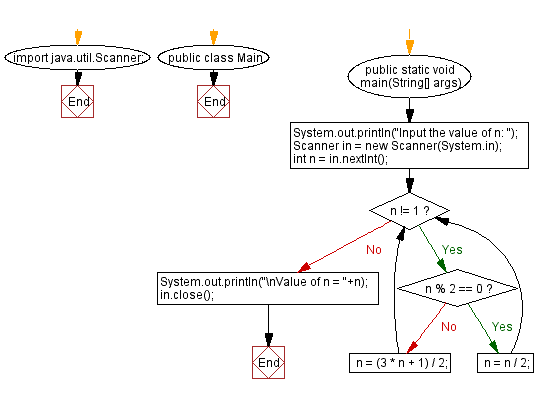﻿ Java: Divide n by 2, repeat the process until n = 1# Java Exercises: Program to start with an integer n, divide n by 2 if n is even or multiply by 3 and add 1 if n is odd, repeat the process until n = 1

## Java Basic: Exercise-86 with Solution

Write a Java program start with an integer n, divide n by 2 if n is even or multiply by 3 and add 1 if n is odd, repeat the process until n = 1.

Sample Solution:

Java Code:

``````import java.util.Scanner;

public class Main {
public static void main(String[] args) {
System.out.println("Input the value of n: ");
Scanner in = new Scanner(System.in);
int n = in.nextInt();
while (n != 1) {
if (n % 2 == 0) {
n = n / 2;
}
else {
n = (3 * n + 1) / 2;
}
}
System.out.println("\nValue of n = "+n);
in.close();
}
}
```
```

If input 5

Sample Output:

```Input the value of n:
9

Value of n = 1
```

Flowchart:What is the difficulty level of this exercise?

Test your Programming skills with w3resource's quiz.

﻿

## Java: Tips of the Day

countOccurrences

Counts the occurrences of a value in an array.

Use Arrays.stream().filter().count() to count total number of values that equals the specified value.

```public static long countOccurrences(int[] numbers, int value) {
return Arrays.stream(numbers)
.filter(number -> number == value)
.count();
}
```

Ref: https://bit.ly/3kCAgLb